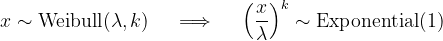﻿WeibullDistribution Class# WeibullDistribution Class

Represents a Weibull distribution.Inheritance Hierarchy

Namespace:  Meta.Numerics.Statistics.Distributions
Assembly:  Meta.Numerics (in Meta.Numerics.dll) Version: 4.1.4Syntax
`public sealed class WeibullDistribution : ContinuousDistribution`

The WeibullDistribution type exposes the following members.Constructors
NameDescriptionWeibullDistribution
Initializes a new Weibull distribution.
TopProperties
NameDescriptionExcessKurtosis
Gets the excess kurtosis of the distribution.
(Inherited from UnivariateDistribution.)Mean
Gets the mean of the distribution.
(Overrides UnivariateDistributionMean.)Median
Gets the median of the distribution.
(Overrides ContinuousDistributionMedian.)Scale
Gets the scale parameter of the distribution.Shape
Gets the shape parameter of the distribution.Skewness
Gets the skewness of the distribution.
(Inherited from UnivariateDistribution.)StandardDeviation
Gets the standard deviation of the distribution.
(Inherited from UnivariateDistribution.)Support
Gets the interval over which the distribution is non-vanishing.
(Overrides ContinuousDistributionSupport.)Variance
Gets the variance of the distribution.
(Overrides UnivariateDistributionVariance.)
TopMethods
NameDescriptionCentralMoment
Computes a central moment of the distribution.
(Overrides ContinuousDistributionCentralMoment(Int32).)Cumulant
Computes a cumulant of the distribution.
(Inherited from UnivariateDistribution.)Equals
Determines whether the specified object is equal to the current object.
(Inherited from Object.)ExpectationValue
Computes the expectation value of the given function.
(Inherited from ContinuousDistribution.)FitToSample
Computes the Weibull distribution that best fits the given sample.GetHashCode
Serves as the default hash function.
(Inherited from Object.)GetRandomValue
Generates a random variate.
(Overrides ContinuousDistributionGetRandomValue(Random).)GetRandomValues
Generates the given number of random variates.
(Inherited from ContinuousDistribution.)GetType
Gets the Type of the current instance.
(Inherited from Object.)Hazard
Computes the hazard function.
(Overrides ContinuousDistributionHazard(Double).)InverseLeftProbability
Returns the point at which the cumulative distribution function attains a given value.
(Overrides ContinuousDistributionInverseLeftProbability(Double).)InverseRightProbability
Returns the point at which the right probability function attains the given value.
(Overrides ContinuousDistributionInverseRightProbability(Double).)LeftProbability
Returns the cumulative probability to the left of (below) the given point.
(Overrides ContinuousDistributionLeftProbability(Double).)ProbabilityDensity
Returns the probability density at the given point.
(Overrides ContinuousDistributionProbabilityDensity(Double).)RawMoment
Computes a raw moment of the distribution.
(Overrides ContinuousDistributionRawMoment(Int32).)RightProbability
Returns the cumulative probability to the right of (above) the given point.
(Overrides ContinuousDistributionRightProbability(Double).)ToString
Returns a string that represents the current object.
(Inherited from Object.)
TopRemarks

The Weibull distribution is a generalized form of the exponential distribution, for which the hazard function is not constant, but instead increases (for shape parameter k > 1) or decreases (for shape parameter k < 1) with x. When the shape parameter is one, the Weibull distribution reduces to the exponential distribution.

In fact, any Weibull distribution can be transformed into a standard exponential distribtuion by a change of variables.The Weibull distribution is commonly used in engineering applications to model the time-to-failure of industrial components.See Also